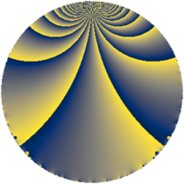# Properties

 Label 120.2.dLevel $120$ Weight $2$ Character orbit 120.d Rep. character $\chi_{120}(109,\cdot)$ Character field $\Q$ Dimension $12$ Newform subspaces $2$ Sturm bound $48$ Trace bound $2$

# Related objects

## Defining parameters

 Level: $$N$$ $$=$$ $$120 = 2^{3} \cdot 3 \cdot 5$$ Weight: $$k$$ $$=$$ $$2$$ Character orbit: $$[\chi]$$ $$=$$ 120.d (of order $$2$$ and degree $$1$$) Character conductor: $$\operatorname{cond}(\chi)$$ $$=$$ $$40$$ Character field: $$\Q$$ Newform subspaces: $$2$$ Sturm bound: $$48$$ Trace bound: $$2$$ Distinguishing $$T_p$$: $$13$$

## Dimensions

The following table gives the dimensions of various subspaces of $$M_{2}(120, [\chi])$$.

Total New Old
Modular forms 28 12 16
Cusp forms 20 12 8
Eisenstein series 8 0 8

## Trace form

 $$12 q + 2 q^{4} - 2 q^{6} + 12 q^{9} + O(q^{10})$$ $$12 q + 2 q^{4} - 2 q^{6} + 12 q^{9} - 10 q^{10} - 20 q^{14} + 2 q^{16} - 4 q^{20} - 2 q^{24} + 4 q^{25} - 28 q^{26} + 12 q^{30} - 32 q^{31} + 24 q^{34} + 2 q^{36} - 16 q^{39} + 22 q^{40} - 8 q^{41} + 44 q^{44} - 4 q^{46} - 12 q^{49} - 2 q^{54} - 16 q^{55} + 52 q^{56} - 22 q^{60} - 46 q^{64} - 24 q^{65} + 20 q^{66} + 32 q^{70} + 32 q^{71} + 36 q^{74} + 12 q^{76} + 32 q^{79} - 12 q^{80} + 12 q^{81} - 4 q^{84} + 40 q^{86} - 40 q^{89} - 10 q^{90} - 4 q^{94} + 64 q^{95} - 42 q^{96} + O(q^{100})$$

## Decomposition of $$S_{2}^{\mathrm{new}}(120, [\chi])$$ into newform subspaces

Label Dim $A$ Field CM Traces $q$-expansion
$a_{2}$ $a_{3}$ $a_{5}$ $a_{7}$
120.2.d.a $6$ $0.958$ 6.0.839056.1 None $$-1$$ $$6$$ $$0$$ $$0$$ $$q+\beta _{3}q^{2}+q^{3}+(-\beta _{1}-\beta _{2})q^{4}+(\beta _{2}+\cdots)q^{5}+\cdots$$
120.2.d.b $6$ $0.958$ 6.0.839056.1 None $$1$$ $$-6$$ $$0$$ $$0$$ $$q-\beta _{2}q^{2}-q^{3}+(\beta _{1}-\beta _{3})q^{4}+(\beta _{1}-\beta _{3}+\cdots)q^{5}+\cdots$$

## Decomposition of $$S_{2}^{\mathrm{old}}(120, [\chi])$$ into lower level spaces

$$S_{2}^{\mathrm{old}}(120, [\chi]) \cong$$ $$S_{2}^{\mathrm{new}}(40, [\chi])$$$$^{\oplus 2}$$